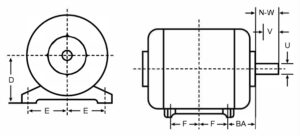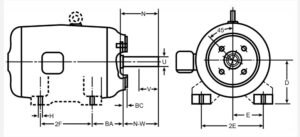# NEMA Standards, Part 2 – Interpreting Frame Sizes

August 9, 2023

Known as NEMA for short, the National Electrical Manufacturers Association, is the United States’ largest electrical equipment manufacturer trade association.

As established in part 1 of our NEMA Standards blog series, uniformity is a must in electrical manufacturing.

At HECO, NEMA’s MG-1 standard for motors and generators is of particular interest when assisting customers in selecting or replacing an electric motor.## By The Numbers

You can determine the motor’s shaft height (D), the distance in inches from the base to the centerline of the shaft, based on the number of digits with the given NEMA frame size.

For example, if there are two digits (X:X), the motor shaft height (D) can be calculated by dividing the two digits by 16.

• D = (both digits) / 16

To find the motor shaft height of a frame size with three digits, divide the first two digits by 4.

• D = (the first two digits) / 4

The rest of the standardized dimensions (E, 2F, BA, N-W, V, and U) can be found in a NEMA Frame Dimensions Table such as The Engineering Toolbox.

The various dimensions are shown below.## An Example

Let’s say you need to replace a 324T electric motor.

Since there are three digits, we drop the third digit, 4, and divide the first two digits by 4.

• 324 becomes 32
• 32 / 4 = 8
• The motor shaft height is 8”

When we look up 324T in a NEMA frame size chart, in addition to D = 8”, we also see:

• E = 6.25”
• F = 5.25”
• BA = 5.25”
• N-W = 4.875”
• U = 2.125”

Using a keyseat dimensions chart with U = 2.125”, we can also determine that R = 1.844”, and S = 0.5”.

The lack of a Z or Y suffix indicates that the shaft and mounting hole dimensions are the same for all motors with the same frame size. Without any physical obstructions surrounding the motor, two 324T motors will mount onto the foundation and couple up the same.

The main terminal box might be in different locations on the motor and may cause issues when trying to connect the motor to incoming power.

## All Systems Go